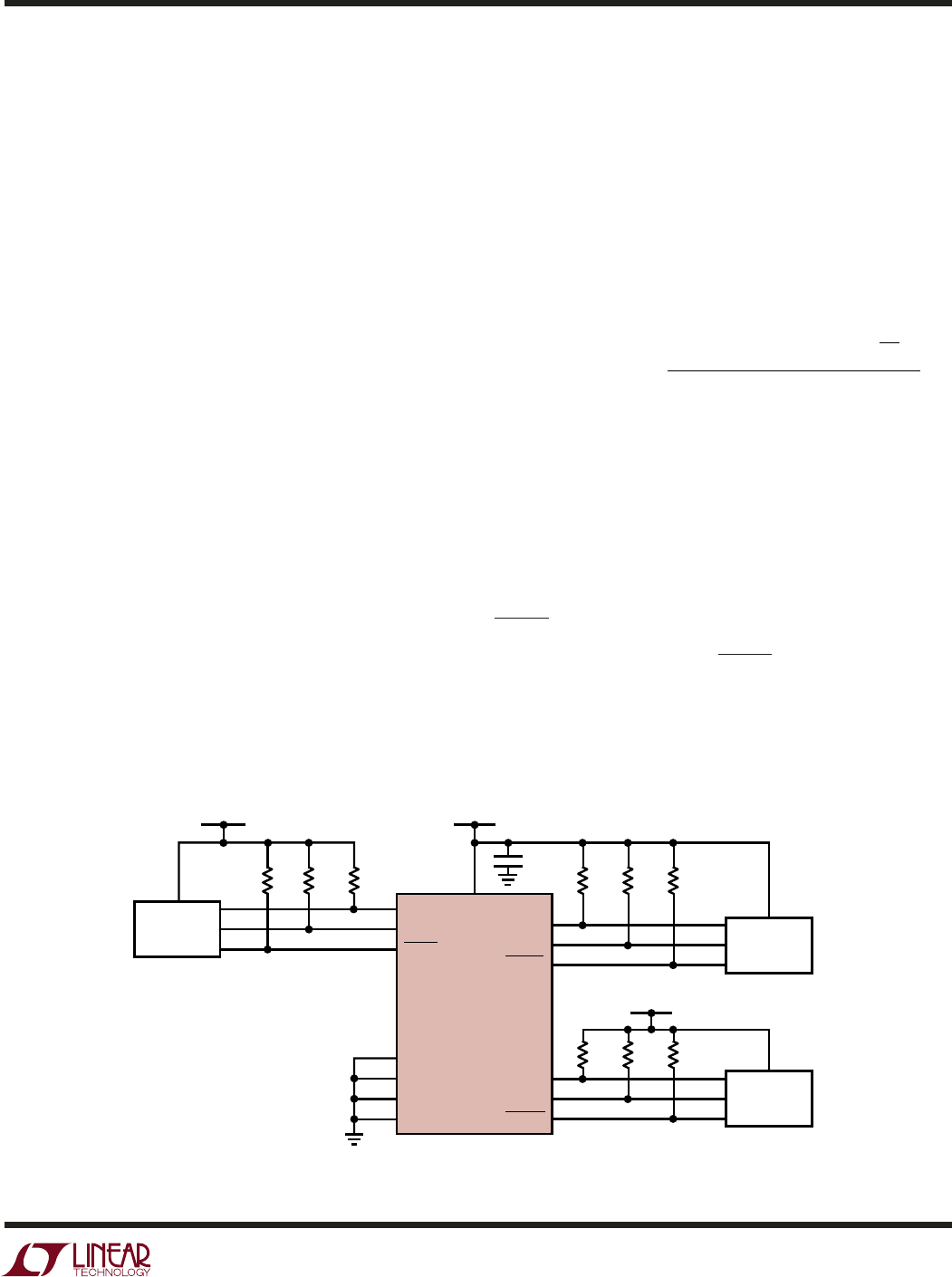# DatasheetLTC4305
15
4305f
Design Example
A typical LTC4305 application circuit is shown in Figure 5.
The circuit illustrates the level-shifting, multiplexer/switch
and capacitance buffering features of the LTC4305. In this
application, the LTC4305 V
CC
voltage and downstream
bus 1 are powered from 3.3V, downstream bus 2 is
powered from 5V, and the upstream bus is powered from
2.5V. The following sections describe a methodology for
choosing the external components in Figure 5.
SDA, SCL Pull-Up Resistor Selection
The pull-up resistors on the SDA and SCL pins must be
strong enough to provide a minimum of 100µA pull-up
current, per the SMBus Specification. In most systems,
the required minimum strength of the pull-up resistors is
determined by the minimum slew requirement to guaran-
tee that the LTC4305’s rise time accelerators are activated
during rising edges. At the same time, the pull-up value
should be kept low to maximize the logic low noise margin
and minimize the offset voltage of the Upstream-Down-
stream Buffer circuitry. The LTC4305 is designed to
function for a maximum DC pull-up current of 4mA. If
multiple downstream channels are active at the same time,
this means that the sum total of the pull-up currents from
these channels must be less than 4mA. At supply voltages
of 2.7V and 5.5V, pull-up resistor values of 10k work well
for capacitive loads up to 215pF and 420pF, respectively.
For larger bus capacitances, refer to equation (1) below.
The LTC4305 works with capacitive loads up to 2nF.
Assume in Figure 5 that the total parasitic bus capacitance
on SDA1 due to trace and device capacitance is 100pF. To
ensure that the boost currents are active during rising
edges, the pull-up resistor must be strong enough to
cause the SDA1 pin voltage to rise at a rate of 0.8V/µs as
the pin voltage is rising above 0.8V. The equation is:
Rk
VV
ns
V
PULL UP MAX
BUSMIN
[]
=
,
(–.)0 8 1250
[]
CpF
BUS
(1)
where V
BUSMIN
is the minimum operating pull-up supply
voltage, and C
BUS
is the bus parasitic capacitance. In our
example, V
BUS1
= V
CC
= 3.3V, and assuming ±10% supply
tolerance, V
BUS1MIN
= 2.97V. With C
BUS
= 100pF,
R
PULL-UP,MAX
= 27.1k. Therefore, we must choose a
pull-up resistor smaller (i.e., stronger pull-up) than 27.1k,
so a 10k resistor works fine.
provide a maximum pull-up current of 3mA, so that the
LTC4305 is capable of holding the pins at logic low
voltages below 0.4V.
APPLICATIO S I FOR ATIO
WUU
U
Figure 5. A Level Shifting Circuit
4305 F05
4
8
9
10
2
3
5
1
15
16
14
13
12
7
R4
10k
C1
0.01µF
R5
10k
R6
10k
R2
10k
R3
10k
R1
10k
SFP
MODULE #1
R7
10k
R8
10k
R9
10k
MICRO-
CONTROLLER
SFP
MODULE #2
V
BACK
= 2.5V
V
BUS2
= 5V
V
CC
= V
BUS1
= 3.3V
SCLIN
SDAIN